# Krylov processes

Krylov processes are the foundation of Krylov methods, they generate bases of Krylov subspaces. Depending on the Krylov subspaces generated, Krylov processes are more or less specialized for a subset of linear problems. The following table summarizes the most relevant processes for each linear problem.

Linear problemsProcesses
Hermitian linear systemsHermitian Lanczos
Square Non-Hermitian linear systemsNon-Hermitian Lanczos – Arnoldi
Least-squares problemsGolub-Kahan – Saunders-Simon-Yip
Least-norm problemsGolub-Kahan – Saunders-Simon-Yip
Saddle-point and Hermitian quasi-definite systemsGolub-Kahan – Saunders-Simon-Yip
Generalized saddle-point and non-Hermitian partitioned systemsMontoison-Orban

### Notation

For a matrix $A$, $A^H$ denotes the conjugate transpose of $A$. It coincides with $A^T$, the transpose of $A$, for real matrices. Define $V_k := \begin{bmatrix} v_1 & \ldots & v_k \end{bmatrix} \enspace$ and $\enspace U_k := \begin{bmatrix} u_1 & \ldots & u_k \end{bmatrix}$.

For a matrix $C \in \mathbb{C}^{n \times n}$ and a vector $t \in \mathbb{C}^{n}$, the $k$-th Krylov subspace generated by $C$ and $t$ is

$$$\mathcal{K}_k(C, t) := \left\{\sum_{i=0}^{k-1} \omega_i C^i t \, \middle \vert \, \omega_i \in \mathbb{C},~0 \le i \le k-1 \right\}.$$$

For matrices $C \in \mathbb{C}^{n \times n} \enspace$ and $\enspace T \in \mathbb{C}^{n \times p}$, the $k$-th block Krylov subspace generated by $C$ and $T$ is

$$$\mathcal{K}_k^{\square}(C, T) := \left\{\sum_{i=0}^{k-1} C^i T \, \Omega_i \, \middle \vert \, \Omega_i \in \mathbb{C}^{p \times p},~0 \le i \le k-1 \right\}.$$$

## Hermitian Lanczos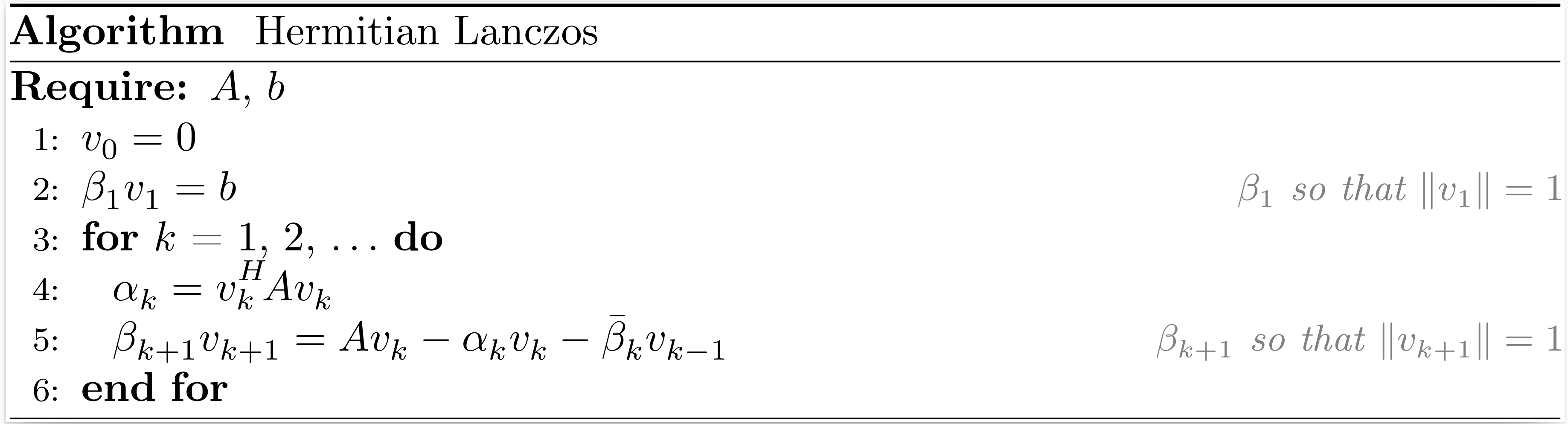After $k$ iterations of the Hermitian Lanczos process, the situation may be summarized as

\begin{align*} A V_k &= V_k T_k + \beta_{k+1,k} v_{k+1} e_k^T = V_{k+1} T_{k+1,k}, \\ V_k^H V_k &= I_k, \end{align*}

where $V_k$ is an orthonormal basis of the Krylov subspace $\mathcal{K}_k(A,b)$,

$$$T_k = \begin{bmatrix} \alpha_1 & \bar{\beta}_2 & & \\ \beta_2 & \alpha_2 & \ddots & \\ & \ddots & \ddots & \bar{\beta}_k \\ & & \beta_k & \alpha_k \end{bmatrix} , \qquad T_{k+1,k} = \begin{bmatrix} T_{k} \\ \beta_{k+1} e_{k}^T \end{bmatrix}.$$$

Note that depending on how we normalize the vectors that compose $V_k$, $T_{k+1,k}$ can be a real tridiagonal matrix even if $A$ is a complex matrix.

The function hermitian_lanczos returns $V_{k+1}$, $\beta_1$ and $T_{k+1,k}$.

Krylov.hermitian_lanczosMethod
V, β, T = hermitian_lanczos(A, b, k)

Input arguments

• A: a linear operator that models an Hermitian matrix of dimension n;
• b: a vector of length n;
• k: the number of iterations of the Hermitian Lanczos process.

Output arguments

• V: a dense n × (k+1) matrix;
• β: a coefficient such that βv₁ = b;
• T: a sparse (k+1) × k tridiagonal matrix.

Reference

source

## Non-Hermitian Lanczos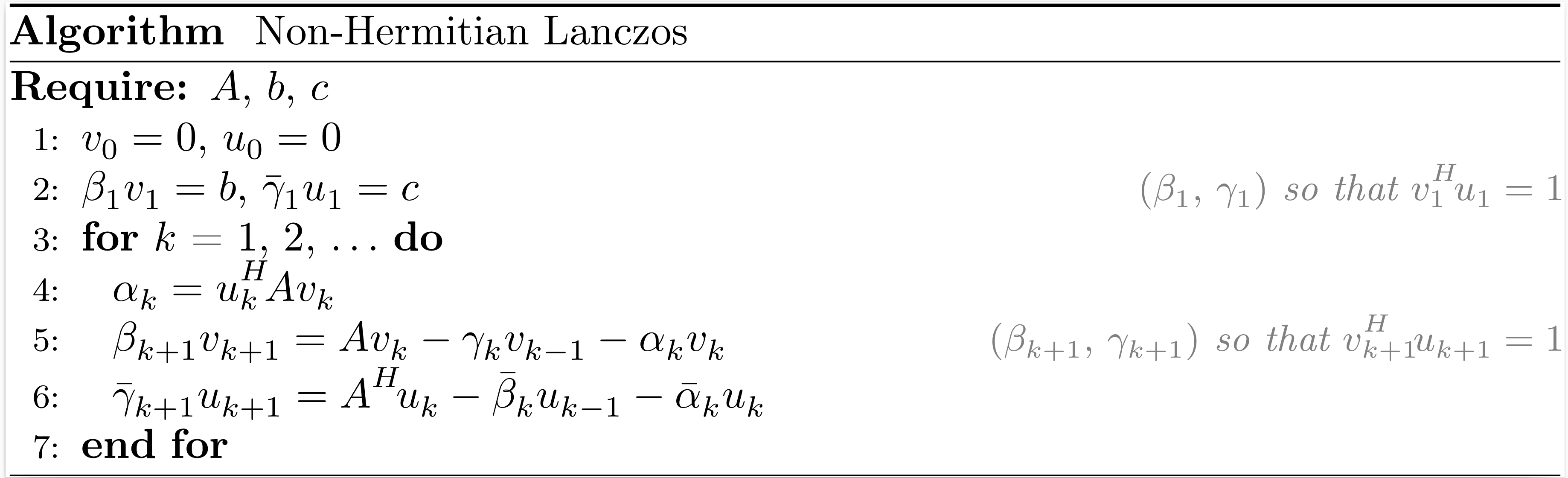After $k$ iterations of the non-Hermitian Lanczos process (also named the Lanczos biorthogonalization process), the situation may be summarized as

\begin{align*} A V_k &= V_k T_k + \beta_{k+1} v_{k+1} e_k^T = V_{k+1} T_{k+1,k}, \\ A^H U_k &= U_k T_k^H + \bar{\gamma}_{k+1} u_{k+1} e_k^T = U_{k+1} T_{k,k+1}^H, \\ V_k^H U_k &= U_k^H V_k = I_k, \end{align*}

where $V_k$ and $U_k$ are bases of the Krylov subspaces $\mathcal{K}_k (A,b)$ and $\mathcal{K}_k (A^H,c)$, respectively,

$$$T_k = \begin{bmatrix} \alpha_1 & \gamma_2 & & \\ \beta_2 & \alpha_2 & \ddots & \\ & \ddots & \ddots & \gamma_k \\ & & \beta_k & \alpha_k \end{bmatrix} , \qquad T_{k+1,k} = \begin{bmatrix} T_{k} \\ \beta_{k+1} e_{k}^T \end{bmatrix} , \qquad T_{k,k+1} = \begin{bmatrix} T_{k} & \gamma_{k+1} e_k \end{bmatrix}.$$$

The function nonhermitian_lanczos returns $V_{k+1}$, $\beta_1$, $T_{k+1,k}$, $U_{k+1}$, $\bar{\gamma}_1$ and $T_{k,k+1}^H$.

Related methods: BiLQ, QMR, BiLQR, CGS and BICGSTAB.

Note

The scaling factors used in our implementation are $\beta_k = |u_k^H v_k|^{\tfrac{1}{2}}$ and $\gamma_k = (u_k^H v_k) / \beta_k$. With these scaling factors, the non-Hermitian Lanczos process coincides with the Hermitian Lanczos process when $A = A^H$ and $b = c$.

Krylov.nonhermitian_lanczosMethod
V, β, T, U, γᴴ, Tᴴ = nonhermitian_lanczos(A, b, c, k)

Input arguments

• A: a linear operator that models a square matrix of dimension n;
• b: a vector of length n;
• c: a vector of length n;
• k: the number of iterations of the non-Hermitian Lanczos process.

Output arguments

• V: a dense n × (k+1) matrix;
• β: a coefficient such that βv₁ = b;
• T: a sparse (k+1) × k tridiagonal matrix;
• U: a dense n × (k+1) matrix;
• γᴴ: a coefficient such that γᴴu₁ = c;
• Tᴴ: a sparse (k+1) × k tridiagonal matrix.

Reference

source

The non-Hermitian Lanczos process can be also implemented without $A^H$ (transpose-free variant). To derive it, we can observe that $\beta_{k+1} v_{k+1} = P_k(A) b~~$ and $\bar{\gamma}_{k+1} u_{k+1} = Q_k(A^H) c~~$ where $P_k$ and $Q_k$ are polynomials of degree $k$. The polynomials are defined from the recursions:

\begin{align*} P_0(A) &= I_n, \\ P_1(A) &= \left(\dfrac{A - \alpha_1 I_n}{\beta_1}\right) P_0(A), \\ P_k(A) &= \left(\dfrac{A - \alpha_k I_n}{\beta_k}\right) P_{k-1}(A) - \dfrac{\gamma_k}{\beta_{k-1}} P_{k-2}(A), \quad k \ge 2, \\ & \\ Q_0(A^H) &= I_n, \\ Q_1(A^H) &= \left(\dfrac{A^H - \bar{\alpha}_1 I_n}{\bar{\gamma}_1}\right) Q_0(A^H), \\ Q_k(A^H) &= \left(\dfrac{A^H - \bar{\alpha}_k I_n}{\bar{\gamma}_k}\right) Q_{k-1}(A^H) - \dfrac{\bar{\beta}_k}{\bar{\gamma}_{k-1}} Q_{k-2}(A^H), \quad k \ge 2. \end{align*}

Because $\alpha_k = u_k^H A v_k$ and $(\bar{\gamma}_{k+1} u_{k+1})^H (\beta_{k+1} v_{k+1}) = \gamma_{k+1} \beta_{k+1}$, we can determine the coefficients of $T_{k+1,k}$ and $T_{k,k+1}^H$ as follows:

\begin{align*} \alpha_k &= \dfrac{1}{\gamma_k \beta_k} \langle~Q_{k-1}(A^H) c \, , \, A P_{k-1}(A) b~\rangle \\ &= \dfrac{1}{\gamma_k \beta_k} \langle~c \, , \, \bar{Q}_{k-1}(A) A P_{k-1}(A) b~\rangle, \\ & \\ \beta_{k+1} \gamma_{k+1} &= \langle~Q_k(A^H) c \, , \, P_k(A) b~\rangle \\ &= \langle~c \, , \, \bar{Q}_k(A) P_k(A) b~\rangle. \end{align*}

## Arnoldi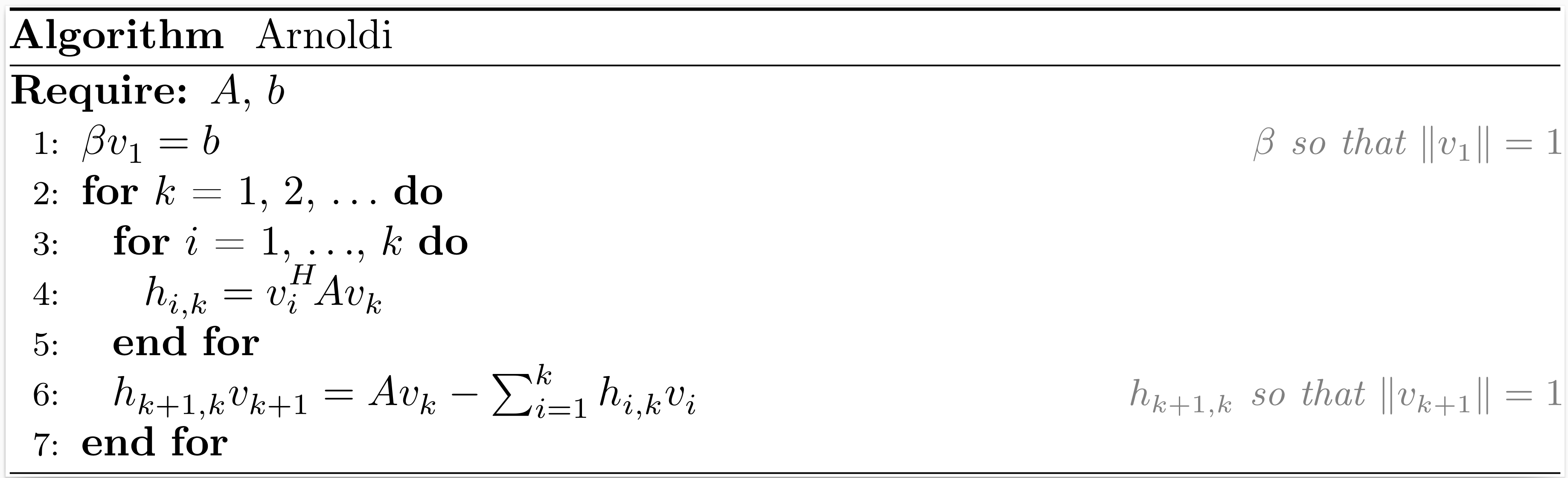After $k$ iterations of the Arnoldi process, the situation may be summarized as

\begin{align*} A V_k &= V_k H_k + h_{k+1,k} v_{k+1} e_k^T = V_{k+1} H_{k+1,k}, \\ V_k^H V_k &= I_k, \end{align*}

where $V_k$ is an orthonormal basis of the Krylov subspace $\mathcal{K}_k (A,b)$,

$$$H_k = \begin{bmatrix} h_{1,1}~ & h_{1,2}~ & \ldots & h_{1,k} \\ h_{2,1}~ & \ddots~ & \ddots & \vdots \\ & \ddots~ & \ddots & h_{k-1,k} \\ & & h_{k,k-1} & h_{k,k} \end{bmatrix} , \qquad H_{k+1,k} = \begin{bmatrix} H_{k} \\ h_{k+1,k} e_{k}^T \end{bmatrix}.$$$

The function arnoldi returns $V_{k+1}$, $\beta$ and $H_{k+1,k}$.

Related methods: DIOM, FOM, DQGMRES, GMRES and FGMRES.

Note

The Arnoldi process coincides with the Hermitian Lanczos process when $A$ is Hermitian.

Krylov.arnoldiMethod
V, β, H = arnoldi(A, b, k; reorthogonalization=false)

Input arguments

• A: a linear operator that models a square matrix of dimension n;
• b: a vector of length n;
• k: the number of iterations of the Arnoldi process.

Keyword argument

• reorthogonalization: reorthogonalize the new vectors of the Krylov basis against all previous vectors.

Output arguments

• V: a dense n × (k+1) matrix;
• β: a coefficient such that βv₁ = b;
• H: a dense (k+1) × k upper Hessenberg matrix.

Reference

source

## Golub-Kahan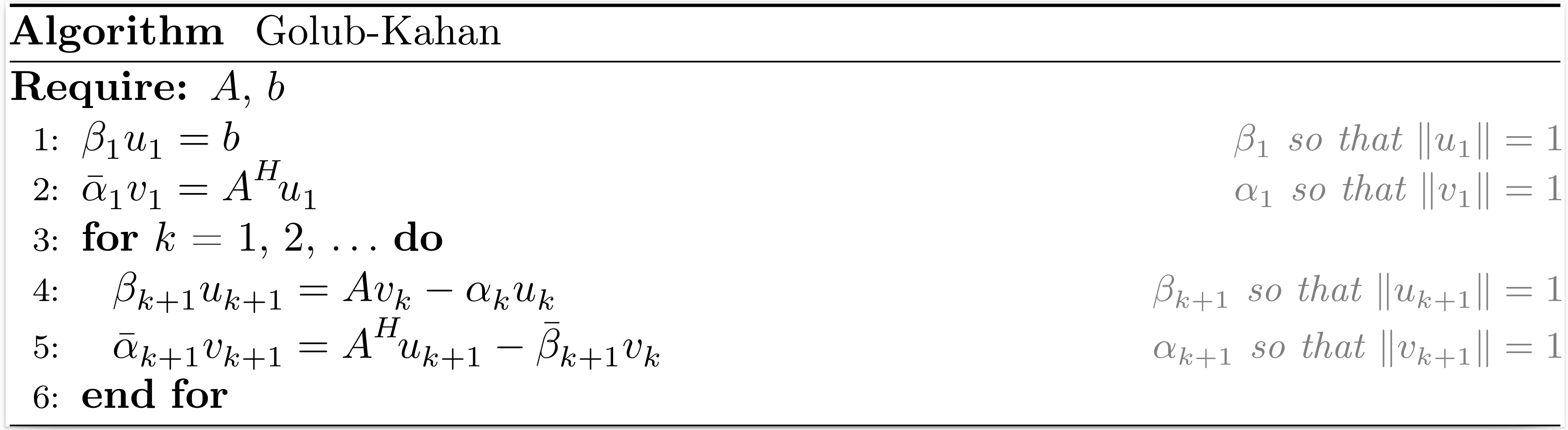After $k$ iterations of the Golub-Kahan bidiagonalization process, the situation may be summarized as

\begin{align*} A V_k &= U_{k+1} B_k, \\ A^H U_{k+1} &= V_k B_k^H + \bar{\alpha}_{k+1} v_{k+1} e_{k+1}^T = V_{k+1} L_{k+1}^H, \\ V_k^H V_k &= U_k^H U_k = I_k, \end{align*}

where $V_k$ and $U_k$ are bases of the Krylov subspaces $\mathcal{K}_k (A^HA,A^Hb)$ and $\mathcal{K}_k (AA^H,b)$, respectively,

$$$L_k = \begin{bmatrix} \alpha_1 & & & \\ \beta_2 & \alpha_2 & & \\ & \ddots & \ddots & \\ & & \beta_k & \alpha_k \end{bmatrix} , \qquad B_k = \begin{bmatrix} \alpha_1 & & & \\ \beta_2 & \alpha_2 & & \\ & \ddots & \ddots & \\ & & \beta_k & \alpha_k \\ & & & \beta_{k+1} \\ \end{bmatrix} = \begin{bmatrix} L_{k} \\ \beta_{k+1} e_{k}^T \end{bmatrix}.$$$

Note that depending on how we normalize the vectors that compose $V_k$ and $U_k$, $L_k$ can be a real bidiagonal matrix even if $A$ is a complex matrix.

The function golub_kahan returns $V_{k+1}$, $U_{k+1}$, $\beta_1$ and $L_{k+1}$.

Related methods: LNLQ, CRAIG, CRAIGMR, LSLQ, LSQR and LSMR.

Note

The Golub-Kahan process coincides with the Hermitian Lanczos process applied to the normal equations $A^HA x = A^Hb$ and $AA^H x = b$. It is also related to the Hermitian Lanczos process applied to $\begin{bmatrix} 0 & A \\ A^H & 0 \end{bmatrix}$ with initial vector $\begin{bmatrix} b \\ 0 \end{bmatrix}$.

Krylov.golub_kahanMethod
V, U, β, L = golub_kahan(A, b, k)

Input arguments

• A: a linear operator that models a matrix of dimension m × n;
• b: a vector of length m;
• k: the number of iterations of the Golub-Kahan process.

Output arguments

• V: a dense n × (k+1) matrix;
• U: a dense m × (k+1) matrix;
• β: a coefficient such that βu₁ = b;
• L: a sparse (k+1) × (k+1) lower bidiagonal matrix.

References

source

## Saunders-Simon-Yip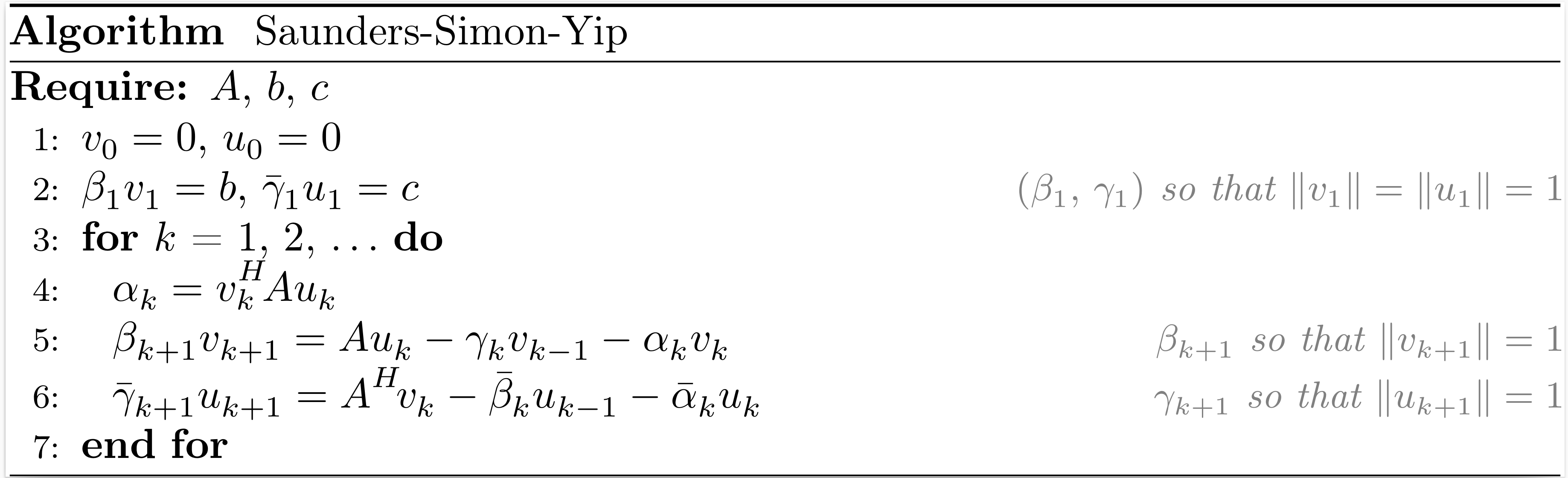After $k$ iterations of the Saunders-Simon-Yip process (also named the orthogonal tridiagonalization process), the situation may be summarized as

\begin{align*} A U_k &= V_k T_k + \beta_{k+1} v_{k+1} e_k^T = V_{k+1} T_{k+1,k}, \\ A^H V_k &= U_k T_k^H + \bar{\gamma}_{k+1} u_{k+1} e_k^T = U_{k+1} T_{k,k+1}^H, \\ V_k^H V_k &= U_k^H U_k = I_k, \end{align*}

where $\begin{bmatrix} V_k & 0 \\ 0 & U_k \end{bmatrix}$ is an orthonormal basis of the block Krylov subspace $\mathcal{K}^{\square}_k \left(\begin{bmatrix} 0 & A \\ A^H & 0 \end{bmatrix}, \begin{bmatrix} b & 0 \\ 0 & c \end{bmatrix}\right)$,

$$$T_k = \begin{bmatrix} \alpha_1 & \gamma_2 & & \\ \beta_2 & \alpha_2 & \ddots & \\ & \ddots & \ddots & \gamma_k \\ & & \beta_k & \alpha_k \end{bmatrix} , \qquad T_{k+1,k} = \begin{bmatrix} T_{k} \\ \beta_{k+1} e_{k}^T \end{bmatrix} , \qquad T_{k,k+1} = \begin{bmatrix} T_{k} & \gamma_{k+1} e_{k} \end{bmatrix}.$$$

The function saunders_simon_yip returns $V_{k+1}$, $\beta_1$, $T_{k+1,k}$, $U_{k+1}$, $\bar{\gamma}_1$ and $T_{k,k+1}^H$.

Related methods: USYMLQ, USYMQR, TriLQR, TriCG and TriMR.

Note

The Saunders-Simon-Yip is equivalent to the block-Lanczos process applied to $\begin{bmatrix} 0 & A \\ A^H & 0 \end{bmatrix}$ with initial matrix $\begin{bmatrix} b & 0 \\ 0 & c \end{bmatrix}$.

Krylov.saunders_simon_yipMethod
V, β, T, U, γᴴ, Tᴴ = saunders_simon_yip(A, b, c, k)

Input arguments

• A: a linear operator that models a matrix of dimension m × n;
• b: a vector of length m;
• c: a vector of length n;
• k: the number of iterations of the Saunders-Simon-Yip process.

Output arguments

• V: a dense m × (k+1) matrix;
• β: a coefficient such that βv₁ = b;
• T: a sparse (k+1) × k tridiagonal matrix;
• U: a dense n × (k+1) matrix;
• γᴴ: a coefficient such that γᴴu₁ = c;
• Tᴴ: a sparse (k+1) × k tridiagonal matrix.

Reference

source

## Montoison-Orban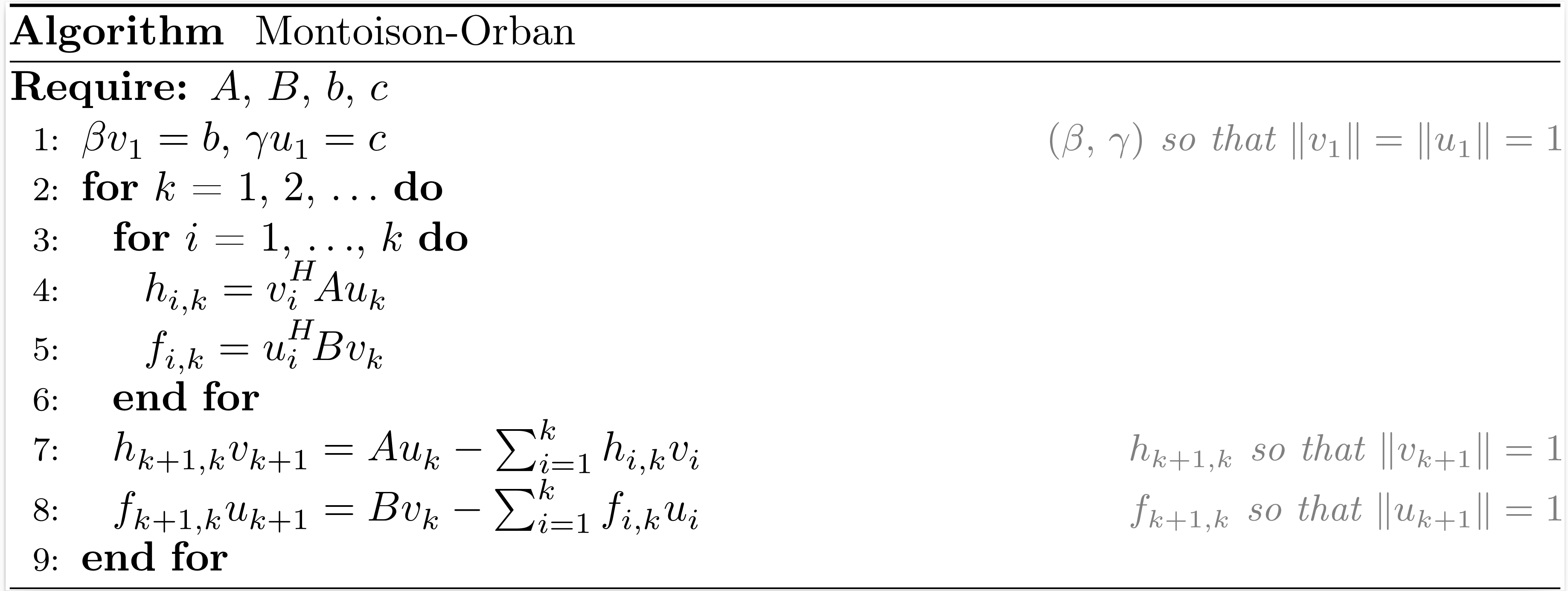After $k$ iterations of the Montoison-Orban process (also named the orthogonal Hessenberg reduction process), the situation may be summarized as

\begin{align*} A U_k &= V_k H_k + h_{k+1,k} v_{k+1} e_k^T = V_{k+1} H_{k+1,k}, \\ B V_k &= U_k F_k + f_{k+1,k} u_{k+1} e_k^T = U_{k+1} F_{k+1,k}, \\ V_k^H V_k &= U_k^H U_k = I_k, \end{align*}

where $\begin{bmatrix} V_k & 0 \\ 0 & U_k \end{bmatrix}$ is an orthonormal basis of the block Krylov subspace $\mathcal{K}^{\square}_k \left(\begin{bmatrix} 0 & A \\ B & 0 \end{bmatrix}, \begin{bmatrix} b & 0 \\ 0 & c \end{bmatrix}\right)$,

$$$H_k = \begin{bmatrix} h_{1,1}~ & h_{1,2}~ & \ldots & h_{1,k} \\ h_{2,1}~ & \ddots~ & \ddots & \vdots \\ & \ddots~ & \ddots & h_{k-1,k} \\ & & h_{k,k-1} & h_{k,k} \end{bmatrix} , \qquad F_k = \begin{bmatrix} f_{1,1}~ & f_{1,2}~ & \ldots & f_{1,k} \\ f_{2,1}~ & \ddots~ & \ddots & \vdots \\ & \ddots~ & \ddots & f_{k-1,k} \\ & & f_{k,k-1} & f_{k,k} \end{bmatrix},$$$$$$H_{k+1,k} = \begin{bmatrix} H_{k} \\ h_{k+1,k} e_{k}^T \end{bmatrix} , \qquad F_{k+1,k} = \begin{bmatrix} F_{k} \\ f_{k+1,k} e_{k}^T \end{bmatrix}.$$$

The function montoison_orban returns $V_{k+1}$, $\beta$, $H_{k+1,k}$, $U_{k+1}$, $\gamma$ and $F_{k+1,k}$.

Related methods: GPMR.

Note

The Montoison-Orban is equivalent to the block-Arnoldi process applied to $\begin{bmatrix} 0 & A \\ B & 0 \end{bmatrix}$ with initial matrix $\begin{bmatrix} b & 0 \\ 0 & c \end{bmatrix}$. It also coincides with the Saunders-Simon-Yip process when $B = A^H$.

Krylov.montoison_orbanMethod
V, β, H, U, γ, F = montoison_orban(A, B, b, c, k; reorthogonalization=false)

Input arguments

• A: a linear operator that models a matrix of dimension m × n;
• B: a linear operator that models a matrix of dimension n × m;
• b: a vector of length m;
• c: a vector of length n;
• k: the number of iterations of the Montoison-Orban process.

Keyword argument

• reorthogonalization: reorthogonalize the new vectors of the Krylov basis against all previous vectors.

Output arguments

• V: a dense m × (k+1) matrix;
• β: a coefficient such that βv₁ = b;
• H: a dense (k+1) × k upper Hessenberg matrix;
• U: a dense n × (k+1) matrix;
• γ: a coefficient such that γu₁ = c;
• F: a dense (k+1) × k upper Hessenberg matrix.

Reference

source